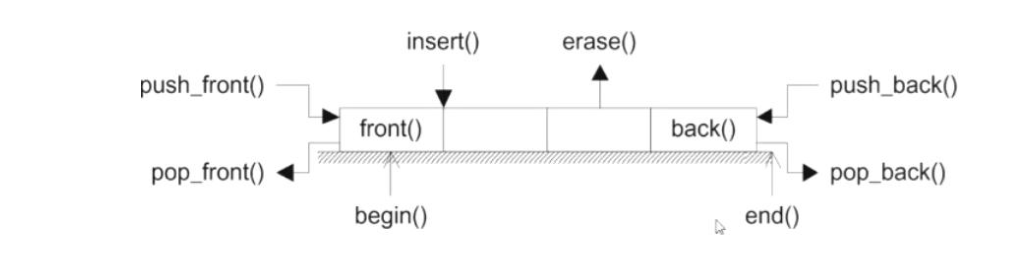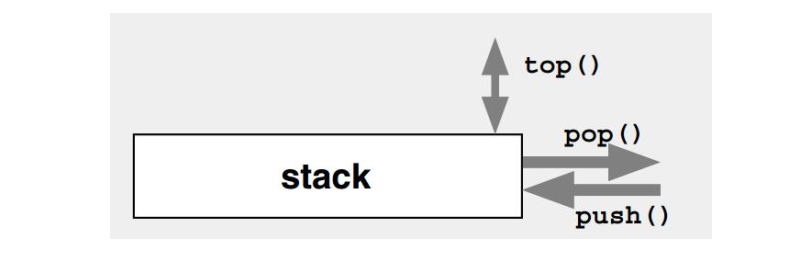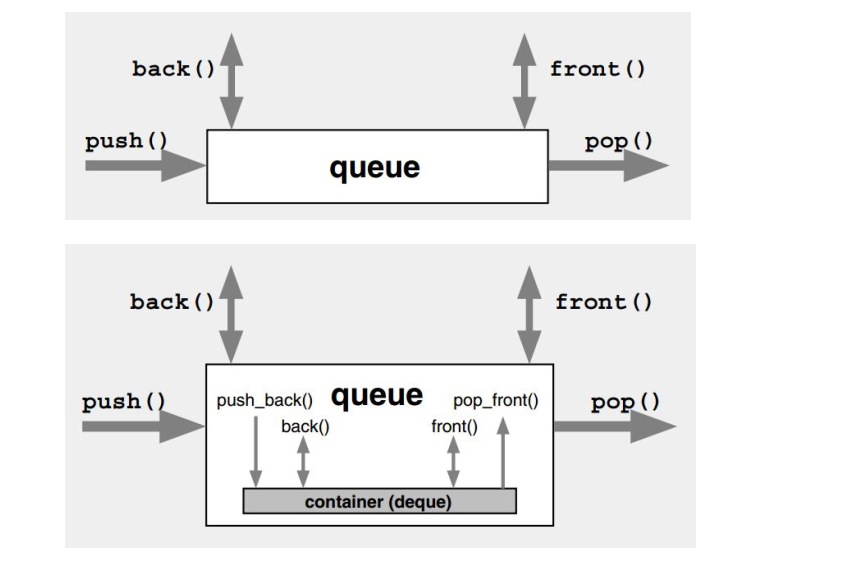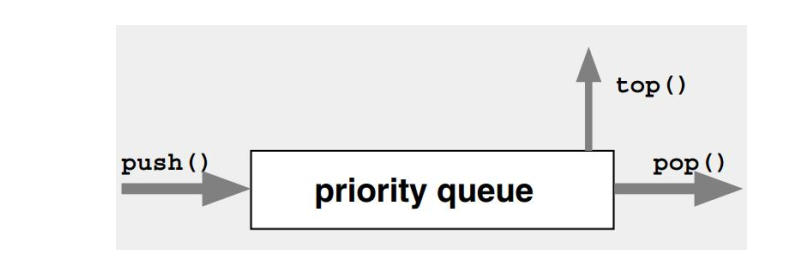# 顺序容器之Dequeue双端队列

## 1、内存结构### 声明及头文件

``````#include <deque>
using namespace std;``````

depue可以模拟出队列和栈的操作，当然我们有更好的方法，请看下文：

## 2、容器适配器

STL 提供了三个容器适配器： queue、 priority_queue、 stack。 这些适配器都是包装了vector、 list、 deque 中某个顺序容器的包装器。 注意： 适配器没有提供迭代器， 也不能同时插入或删除多个元素。

### stack``````#include <iostream>
#include <stack>
using namespace std;
int main()
{
stack<int> si;
cout<<si.size()<<endl;
for(int i=0; i<10; i++)
{
si.push(i);//push数据
}
cout<<si.size()<<endl;
while(!si.empty())
{
cout<<si.top();//使用top
si.pop();//pop数据
}
cout<<endl<<si.size()<<endl;
return 0;
}``````

### queue``````#include <iostream>
#include <queue>
using namespace std;
int main()
{
queue<int> qi;
cout<<qi.size()<<endl;
for(int i=0; i<10; i++)
{
qi.push(i);
}
while(!qi.empty())
{
cout<<qi.front()<<" ";//用front
qi.pop();
}
cout<<endl<<qi.size()<<endl;
return 0;
}``````

### 优先队列 priority Queue``````namespace std {
template <typename T,
typename Container = vector<T>,
typename Compare = less<typename Container::value_type>>
class priority_queue;
}``````

``````#include <queue>
#include <cstring>
#include <cstdio>
using namespace std;
//结构体
struct Node
{
Node(int nri, char *pszName)
{
priority = nri;
strcpy(szName, pszName);
}
char szName;
int priority;
};
//结构体的比较方法 改写 operator()
class NodeCmp
{
public:
bool operator()(const Node &na, const Node &nb)
{
if (na.priority != nb.priority)
return na.priority > nb.priority;
else
return strcmp(na.szName, nb.szName) < 0;
}
};
void PrintfNode(const Node &na)
{
printf("%s %d\n", na.szName, na.priority);
}
int main()
{
//优先级队列默认是使用 vector 作容器， 底层数据结构为堆。
priority_queue<Node, vector<Node>, NodeCmp> a;
//有 5 个人进入队列
a.push(Node(5, "abc"));
a.push(Node(3, "bac"));
a.push(Node(1, "cba"));
a.push(Node(5, "aaa"));
//队头的 2 个人出队
PrintfNode(a.top());
a.pop();
PrintfNode(a.top());
a.pop();
printf("--------------------\n");
//再进入 3 个人
a.push(Node(2, "bbb"));
a.push(Node(2, "ccc"));
a.push(Node(3, "aaa"));
//所有人都依次出队
while (!a.empty())
{
PrintfNode(a.top());
a.pop();
}
//2 ccc 2 bbb 3 aaa 5abc 5aaa
return 0;
}
``````
Last modification：October 6th, 2019 at 02:34 pm